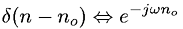Equations > Signal Processing > Fourier Transform Pairs > Discrete-Time Fourier transform of shifted delta

### Discrete-Time Fourier transform of shifted deltaLatex Code:

MathML Code:

 $\delta \left(n-{n}_{o}\right)⇔{e}^{-j\omega {n}_{o}}$

MathType 5.0: